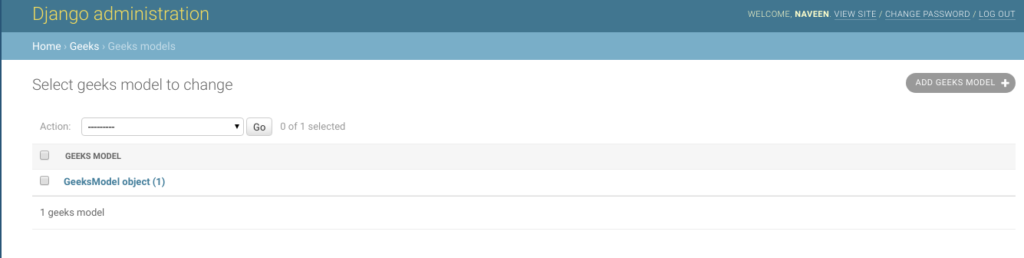# IntegerField – Django Models

## IntegerField – Django Models

IntegerField is a integer number represented in Python by a int instance. This field is generally used to store integer numbers in the database. The default form widget for this field is a NumberInput when localize is False or TextInput otherwise. It supports values from -2147483648 to 2147483647 are safe in all databases supported by Django.It uses MinValueValidator and MaxValueValidator to validate the input based on the values that the default database supports.

Syntax:

`field_name = models.IntegerField(**options)`

## Django Model IntegerField Explanation

Illustration of IntegerField using an Example. Consider a project named `geeksforgeeks` having an app named `geeks`.

Refer to the following articles to check how to create a project and an app in Django.

Enter the following code into `models.py` file of geeks app.

 `from` `django.db ``import` `models` `from` `django.db.models ``import` `Model` `# Create your models here.` ` ` `class` `GeeksModel(Model):` `    ``geeks_field ``=` `models.IntegerField()`

Add the geeks app to `INSTALLED_APPS`

 `# Application definition` ` ` `INSTALLED_APPS ``=` `[` `    ``'django.contrib.admin'``,` `    ``'django.contrib.auth'``,` `    ``'django.contrib.contenttypes'``,` `    ``'django.contrib.sessions'``,` `    ``'django.contrib.messages'``,` `    ``'django.contrib.staticfiles'``,` `    ``'geeks'``,` `]`

Now when we run `makemigrations` command from the terminal,

`Python manage.py makemigrations`

A new folder named migrations would be created in `geeks` directory with a file named `0001_initial.py`

 `# Generated by Django 2.2.5 on 2019-09-25 06:00` ` ` `from` `django.db ``import` `migrations, models` ` ` `class` `Migration(migrations.Migration):` ` ` `    ``initial ``=` `True` ` ` `    ``dependencies ``=` `[` `    ``]` ` ` `    ``operations ``=` `[` `        ``migrations.CreateModel(` `            ``name ``=``'GeeksModel'``,` `            ``fields ``=``[` `                ``(``'id'``, ` `                  ``models.AutoField(` `                  ``auto_created ``=` `True``,` `                  ``primary_key ``=` `True``,` `                  ``serialize ``=` `False``, ` `                  ``verbose_name ``=``'ID'` `                ``)),` `                ``(``'geeks_field'``, ` `                  ``models.IntegerField()),` `            ``],` `        ``),` `    ``]`

Now run,

`Python manage.py migrate`

Thus, an` geeks_field `IntegerField is created when you run migrations on the project. It is a field to store a integer numbers.

## How to use IntegerField ?

IntegerField is used for storing a integer number represented in Python by a int instance. To know more about int, visit Python | int() function. Let’s try to save a flating number in FlatField.

 `# importing the model` `# from geeks app` `from` `geeks.models ``import` `GeeksModel` ` ` `# creating an instance of` `# int` `d ``=` `int``(``2189``)` ` ` `# creating a instance of ` `# GeeksModel` `geek_object ``=` `GeeksModel.objects.create(geeks_field ``=` `d)` `geek_object.save()`

Now let’s check it in admin server. We have created an instance of GeeksModel.## Field Options

Field Options are the arguments given to each field for applying some constraint or imparting a particular characteristic to a particular Field. For example, adding an argument `null = True` to IntegerField will enable it to store empty values for that table in relational database.
Here are the field options and attributes that an IntegerField can use.

Field Options Description
Null If True, Django will store empty values as NULL in the database. Default is False.
Blank If True, the field is allowed to be blank. Default is False.
db_column The name of the database column to use for this field. If this isn’t given, Django will use the field’s name.
Default The default value for the field. This can be a value or a callable object. If callable it will be called every time a new object is created.
help_text Extra “help” text to be displayed with the form widget. It’s useful for documentation even if your field isn’t used on a form.
primary_key If True, this field is the primary key for the model.
editable If False, the field will not be displayed in the admin or any other ModelForm. They are also skipped during model validation. Default is True.
error_messages The error_messages argument lets you override the default messages that the field will raise. Pass in a dictionary with keys matching the error messages you want to override.
help_text Extra “help” text to be displayed with the form widget. It’s useful for documentation even if your field isn’t used on a form.
verbose_name A human-readable name for the field. If the verbose name isn’t given, Django will automatically create it using the field’s attribute name, converting underscores to spaces.
validators A list of validators to run for this field. See the validators documentation for more information.
Unique If True, this field must be unique throughout the table.

Last Updated on March 17, 2022 by admin

## Sort the words in lexicographical order in PythonSort the words in lexicographical order in Python

Sort the words in lexicographical order in Python Given a strings, we need to sort

## Python program to find the sum of all items in a dictionaryPython program to find the sum of all items in a dictionary

Python program to find the sum of all items in a dictionary Given a dictionary

## Classifying data using Support Vector Machines(SVMs) in PythonClassifying data using Support Vector Machines(SVMs) in Python

Classifying data using Support Vector Machines(SVMs) in Python Introduction to SVMs: In machine learning, support

## Normal Equation in Linear RegressionNormal Equation in Linear Regression

ML | Normal Equation in Linear Regression Normal Equation is an analytical approach to Linear Regression

## Python String isspace() MethodPython String isspace() Method

Python String isspace() Method Python String isspace() is a built-in method used for string handling. The isspace()

## Python – Check if all the values in a list that are greater than a given valuePython – Check if all the values in a list that are greater than a given value

Python | Check if all the values in a list that are greater than a

## Conway’s Game Of Life (Python Implementation)Conway’s Game Of Life (Python Implementation)

Conway’s Game Of Life (Python Implementation) Conways’s Game Of Life is a Cellular Automation Method

## Find all the numbers in a string using regular expression in PythonFind all the numbers in a string using regular expression in Python

Find all the numbers in a string using regular expression in Python Given a string str containing